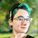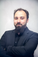## CMS DevelopmentParticipant

## Can't get two variables to divide

For some reason i can do every operation except for diving theese 2 variables. I've tried every aleternative to round them and still nothing changes. The code first sum's up an array with numbers it got from a for loop. Heres the code:

{% set number1 = list_number|sum|round(1) %}
{% set number = numbers|sum|multiply(10)|round(1) %}

{{ number / number1 }}

and this is the error it comes up with when i try to publish:

1. Error:Cannot resolve property

Any help would be appreciated
8 Replies 8Key Advisor | Elite Partner

## Can't get two variables to divide

@AKoprivec If you add {{ number }} and {{ number1 }} at the very end of the code what do the variables return (note that you can't divide by zero, so if they're returning the default value it's going to error out)?

If this answer solved your question, please mark it as the solution!### Alyssa Wilie

#### Web Developer at LyntonWeb

Kickstart your success on HubSpot's CMS with a theme by the HubSpot experts at LyntonParticipant

## Can't get two variables to divide

They both return a number, all operations besides / and // work completly fine on both numbers, and it doesn't show any red alerts saying it can't devide with 0 like it does if i do {{number}}/0 instead it just gives an orange alert on that line.Recognized Expert | Diamond Partner

## Can't get two variables to divide

Hi @AKoprivec,

could you please provide the whole code?

Also if the numbers are set in the module - do you have set a default number?

best,

AntonParticipant

## Can't get two variables to divide

Hi @Anton
Yes ofcourse, here is the whole code:

``````{% set list_variable = request_contact.list_memberships %}
{% set numbers = [] %}
{% set list_number = [] %}

{% for i in module.questions %}
{% for list in i.lists %}
{% if list.list_id in list_variable %}
{% do numbers.append(list.list_value) %}
{% endif %}
{% endfor %}
{% do list_number.append(1) %}
{% endfor %}

{% set number1 = list_number|sum|round(0) %}
{% set number = numbers|sum|multiply(10)|round(0) %}``````

The module gets all of the contacts lists and checks which of them are the same as the ones input into fields and then assigns them the corresponding value. The top part of the script should work fine, as the end result is 2 numbers. Every single operation between them works just fine except for / and //.  And yes all the modules have a set default number of 0 (both list_id and list_value).

thank you for your help,

best,

anžeRecognized Expert | Diamond Partner

## Can't get two variables to divide

Hi @AKoprivec

what are list_number and numbers? Are they set before your calculation?

You have to define them somehow. For example like this:

``````{% set list_number = 2 %}
{% set numbers = 5 %}
{% set number1 = list_number|sum|round(1) %}
{% set number = numbers|sum|multiply(10)|round(1) %}

{{ number / number1 }}``````

best,

AntonParticipant

## Can't get two variables to divide

Hi Anton,

yes they are both defined before as arrays

{% set numbers = [] %}
{% set list_number = [] %}

but since they are converted with |sum it should be fine?

Best
AnzeRecognized Expert | Diamond Partner

## Can't get two variables to divide

Yes,

but if they're empty there is nothing "to sum up". And this means that the outcome can't be calculated.

You can populate it with (contact) properties, module functions or whatever you like - but the array can't be empty

best,

AntonParticipant

## Can't get two variables to divide

Yes the array isnt empty, just using {{ number }} and {{ number1 }}
displays both numbers (assuming as integers since other operations work )
just fine. Is there maybe any other way to run variables out of the for
loop without appending the numbers to an array

best,
Anže